Transient Response of Inductor-Resistor-Capacitor (LRC) Circuits
Natural Frequencies

The Transient Response of Series LRC Circuit

 Here is a source-free Series LRC Circuit: By applying KVL we can generate a 2nd-order Differential Equation. L di/dt + R i + 1/C ∫i dt + Vo = 0 Now differentiate this equation to eliminate the integral: L d²i/dt² + R di/dt + i/C = 0 Let i = Aest, therefore di/dt = si and d²i/dt² = s²i We now have the following equation: L s²i + R si + i/C = 0    →   i [L s² + R s + 1/C] = 0 Since i ≠ 0, then we use L s² + R s + 1/C = 0 to find s Using the quadratic formula: s = [ -b ± √(b² - 4ac) ] / 2a ,    and letting  a=L, b=R, and c=1/C We get the following equation: s = [ -R ± √(R² - 4L/C) ] / 2L Note there are two roots, s1 and s2. These roots are called the natural frequencies. s1, s2 = -R/2L ± √[ (R/2L)² - 1/LC ]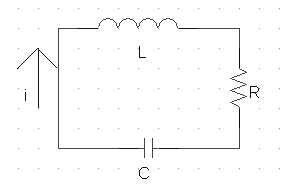For any series LRC circuit, the following equation can be used to find s1 and s2: s1, s2 = -R/2L ± √ [ (R/2L)² - 1/LC ] Note: How s1 and s2 are used is shown in another section.

Top of Page

The Transient Response of Parallel LRC Circuits

 Here is a source-free Parallel LRC Circuit: By applying KCL we can generate a 2nd-order Differential Equation. C dv/dt + 1/L ∫v dt + Io + V/R = 0 Now differentiate this equation to eliminate the integral: C d²v/dt² + v/L + 1/R dv/dt = 0 Let v(t) = Aest, therefore dv/dt = s v(t) and d²v/dt² = s² v(t) Substituting v(t), we get the following equation: C s²v + 1/R sv + v/L = 0    →   v [C s² + 1/R s + 1/L] = 0 Since v(t) ≠ 0, then we use C s² + 1/R s + 1/L = 0 to find s Using the quadratic formula: s = [ -b ± √(b² - 4ac) ] / 2a ,    and letting  a=C, b=1/R, and c=1/L We get the following equation: s = [ -1/R ± √(1/R² - 4C/L) ] / 2C Note there are two roots, s1 and s2. These roots are called the natural frequencies. s1, s2 = -1/(2RC) ± √[ (1/2RC)² - 1/LC ]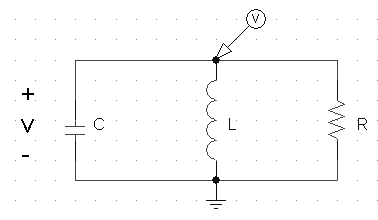For any parallel LRC circuit, the following equation can be used to find s1 and s2: s1, s2 = -1/(2RC) ± √[ (1/2RC)² - 1/LC ] Note: How s1 and s2 are used is shown in another section.

Summary

When solving for the roots of the characteristic equation,
s1 and s2 can fall into one of the following three categories:

 The roots can be both real and distinct. This occurs when the discriminant is positive. s1 ≠ s2 The roots can be complex numbers. This occurs when the discriminant is negative. s1 = α + jβ and s2 = α - jβ The roots can be identical. This occurs when the discriminant is zero. s1 = s2

This table indicates the equation form to use for i(t) or v(t):

 s1 ≠ s2 V(t) = A es1t + B es2t     where A and B are constants. Over-damped Case s1 = α + jβ and s2 = α - jβ V(t) = eαt [ A cosβt + B sinβt ]     where A and B are constants. Under-damped Case s1 = s2 = s V(t) = (A + Bt) est     where A and B are constants. Critically-damped Case

Top of Page

Underdamped second-order circuits

 Here is a series LRC circuit. Therefore to find the natural/transient response, we apply the series formula: s1, s2 = -R/2L ± √ [ (R/2L)² - 1/LC ] s1, s2 = -100/2m ± √ [ (100/2m)² - 1/10-12 ] s1, s2 = -50,000 ± √ [ (25 × 108 - 1012 ] s1 = -50,000 + j (3 × 106) s2 = -50,000 - j (3 × 106) Note that the roots are Complex.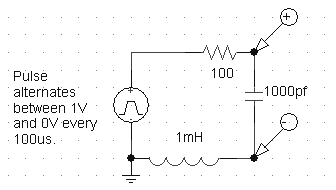Therefore the natural response is: V(t) = e-50,000t [A cos (3×106)t + B sin (3×106)t] (Note that A and B are constants which are found with initial conditions.) V(t) is sinusoidal, therefore we say that the natural response "rings" or resonates. For this reason, the response is Underdamped. Here is the underdamped response:    →Note that the natural response for any voltage or current in the circuit above
has the same form:

X(t) = e-50,000t [A cos (3×106)t + B sin (3×106)t]

The constants A and B are what distinguishes one equation from another.

Does it make sense that every voltage and current have the same mathematical form?

Yes, because if you mathematically manipulate the above equation (through addition,
multiplication integration, differentiation, etc.) you still have the same equation
with the exception of A and B changing.

Top of Page

Overdamped second-order circuits

 Here is another series LRC circuit. Therefore to find the natural/transient response, we apply the series formula: s1, s2 = -R/2L ± √ [ (R/2L)² - 1/LC ] s1, s2 = -100/(0.2m) ± √ [ (100/(0.2m))² - 1/(0.01n) ] Note that m = 10-3   and   n = 10-9 s1, s2 = -500,000 ± √ [ (500,000)² - (100 × 109) ] s1, s2 = -500,000 ± √ [ 15 × 1010 ] s1, s2 = -500,000 ± 387,000 s1 = -887k   and   s2 = -113k Note that k=1000 Note that the two roots are both real, but distinct from each other.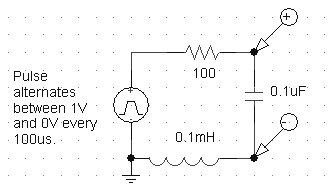Therefore the natural response is: V(t) = Ae-887kt + Be-113kt (Note that A and B are constants which are found with initial conditions.) The response is Overdamped. Here is the overdamped response:    →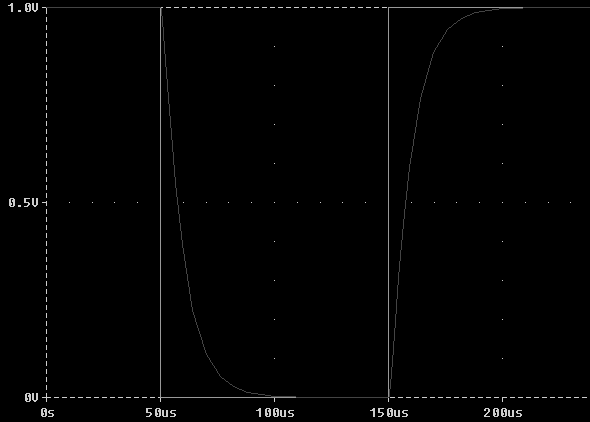Note that the natural response for any voltage or current in the circuit above
has the same form:

X(t) = Ae-887,000t + Be-113,000t

The constants A and B are what distinguishes one equation from another.
A and B are found with initial conditions. (See below.)

Top of Page

Critically Damped second-order circuits

 Here is another series LRC circuit. Therefore to find the natural/transient response, we apply the series formula: s1, s2 = -R/2L ± √ [ (R/2L)² - 1/LC ] s1, s2 = -100/0.2m ± √ [ (100/0.2m)² - 1/4p ] Note p=10-12 s1, s2 = -500,000 ± √ [ (500,000)² - 0.25 × 1012 ] s1, s2 = -500,000 ± √ [ 25 × 1010 - 0.25 × 1012 ] s1, s2 = -500,000 ± √ [ 0 ] s1, s2 = -500,000 Note that s1 and s2 are identical. Therefore this circuit is critically damped.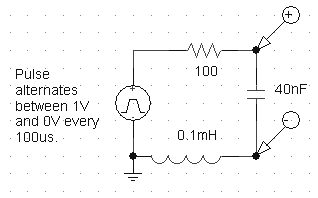Therefore the natural response is: V(t) = Ae-500kt + Bte-500kt (Note that A and B are constants which are found with initial conditions.) The response is critically damped. Here is the critically damped response:    →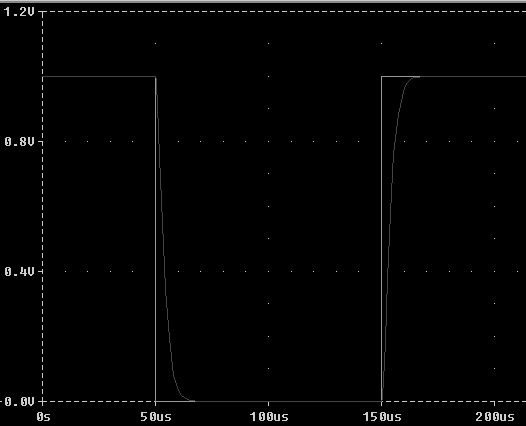Summary

Look carefully at the three responses. Note that the underdamped response falls quickly to zero
but it then 'overshoots' zero. The response resonates (or rings) around zero. After some time,
the response dies out, similar to a bell which eventually stops ringing.

Now compare the overdamped response with the critically damped response. Note that the overdamped
response is 'slow' compared to the critically damped response. The critically damped response
represents the fastest the circuit can decay to zero without overshooting zero.

## Study Problems

After clicking on the following link enter 8-1 for the problem and 1 for the step:
Study Problem 8-1

Top of Page

Finding the constants A and B

To find A and B we may need to use ic = C dv/dt and VL = L di/dt

 Assume the following Natural Response: V(t) = Ae-4t + Be-t Also assume you are given the following information about the circuit: V(t=0) = 4    and    dV/dt(t=0) = 11 Note that two Initial Conditions are needed because there are two unknowns, A and B. We now apply the initial conditions to find A and B: V(t=0) = 4 = Ae0 + Be0 4 = A + B    EQN 1 Using the other initial condition: dV/dt = -4Ae-4t - Be-t dV/dt(t=0) = 11 = -4Ae0 - Be0 11 = -4A - B    EQN 2 Substitute (4 - A) for B in EQN 2: 11 = -4A - (4 - A) 15 = -3A A = -5 therefore B = 9 The Natural Response is: V(t) = -5e-4t + 9e-t Assume the following Natural Response: i(t) = e-2t(A cos3t + B sin3t) Also assume you are given the following information about the circuit: i(t=0) = 3    and    di/dt(t=0) = 6 We now apply the initial conditions to find A and B: i(t=0) = 3 = e0(A cos0 + B sin0) 3 = (A cos0) = A × 1 = A   EQN 1 di/dt = -2e-2t(A cos3t + B sin3t) + e-2t(-3A sin3t + 3B cos3t) di/dt(t=0) = 6 = -2e0(A cos0 + B sin0) + e0(-3A sin0 + 3B cos0) di/dt(t=0) = 6 = -2(A) + (3B)   EQN 2 Plug A=3 into EQN 2: 6 = -2(3) + 3B 12 = 3B B = 4 The Natural Response is: i(t) = e-2t(3 cos3t + 4 sin3t) Assume the following Natural Response: V(t) = (A + Bt)e-3t Also assume you are given the following information about the circuit: V(t=0) = 4   &   dV/dt(t=0) = 8 We now apply the initial conditions to find A and B: V(0) = 4 = (A + 0)e0 = A A = 4  EQN 1 dV/dt = Be-3t - 3(A + Bt)e-3t dV/dt(t=0) = Be0 - 3(A + 0)e0 dV/dt(t=0) = 8 = B - 3A  EQN 2 8 = B -3(4) 8 + 12 = B B = 20 The Natural Response is: V(t) = (4 + 20t)e-3t Assume there is a Forced Response. This will get added to the Natural Response. Assume the following Complete Response. i(t) = Ae-4t + Be-t + 20 Note that the 20 is the forced response - i.e. what is left after the transient response has died out. Also assume you are given the following information about the circuit: i(t=0) = 20    and    di/dt(t=0) = 15 We now apply the initial conditions to find A and B: i(t=0) = 20 = A + B + 20 A = -B    EQN 1 di/dt = -4Ae-4t - Be-t di/dt(t=0) = -4Ae0 - Be0 di/dt(t=0) = 15 = -4A - B    EQN 2 15 = -4A - (-A) 15 = -3A A = -5 B = +5 The Complete Response is: i(t) = -5e-4t + 5e-t + 20

Top of Page

Steps for finding the Complete Response of LRC Circuits

Use these Steps when finding the Complete Response for a 2nd-order Circuit:

• Step 1: First examine the switch to see if it is opening or closing and at what time.

• Step 2: Next draw the circuit right before the switch moves. You will probably assume
steady state at this time but not always. The problem needs to tell you to assume steady state.

• Step 3: Find all voltages and currents that can not change instantaneously when the switch moves.
In other words, Find voltages across all capacitors and currents through all inductors!

• Step 4: Now draw the circuit right after the switch moves. Label the circuit with all the
capacitor voltages and inductor currents you found in step 3.

• Step 5: Now you are ready to find your initial conditions. Analyze the circuit to find the
initial conditions of what it is you are solving for. You may need to use the equations: VL = L di/dt
and Ic = C dV/dt in order to find the second initial condition.

dV/dt(t=0) = Ic(t=0)/C  ←This is the derivative of the voltage across a capacitor at t=0.

di/dt(t=0) = VL(t=0)/L  ←This is the derivative of the current through an inductor at t=0.

• Step 6: Next you will find the natural frequencies, s1 and s2. To do this 'kill' all forcing functions.
Make all independent voltage sources 'shorts' and all current sources 'opens'. Remember that the transient response is the circuit's
response to energies stored in storage elements, so we need to remove forcing functions to find this.
Recall that every voltage and current will have the same natural frequencies.
Using the table above you can choose the correct equation form based on s1 and s2.

• Step 7: Now we need to find the forced response.
The forced response is the state of the circuit after the switch has moved AND after
the transient response has died-off. To find the forced response assume Steady State, i,e, t>>>0.

Find the final resting value (forced response - VF) of whatever it is you are solving for.

• Step 8: You should now add the natural response and the forced response.

Your equation should look like one of the three following:

x(t) = A es1t + B es2t + xF
x(t) = eαt [ A cosβt + B sinβt ] + xF
x(t) = (A + Bt) est + xF

To find the unknowns 'A' and 'B' you will apply the initial conditions to
the equation. Usually the initial condition is the value at t=0.

• Step 9: Follow the examples shown in the last section for finding A and B.

• Step 10:  Using your equation for v(t) or i(t), you can find other things (voltages, currents,
power, etc.) using KVL, KCL, and Ohm's Law.

Top of Page

Study Problems

The following problems will follow the steps above to find the Complete Response of
second order circuits.

## Study Problems

After clicking on the following link enter 8-2 for the problem and 1 for the step:
Study Problem 8-2
After clicking on the following link enter 8-3 for the problem and 1 for the step:
Study Problem 8-3

Top of Page

Back To Index